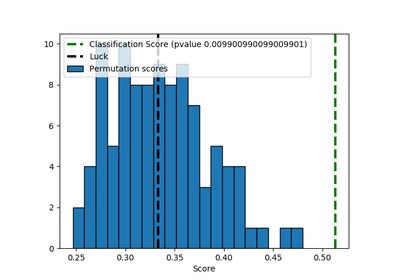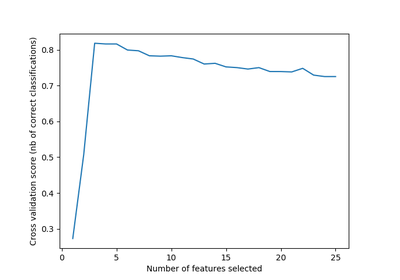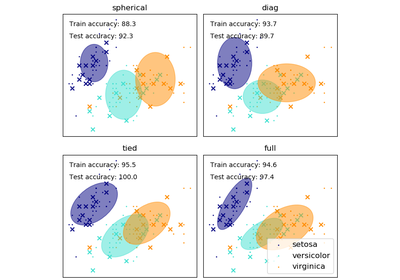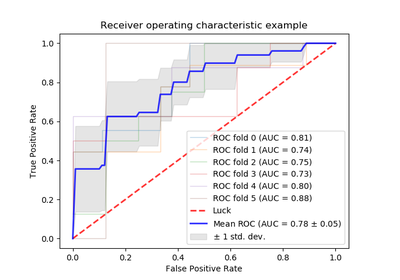# `sklearn.model_selection`.StratifiedKFold¶

class `sklearn.model_selection.``StratifiedKFold`(n_splits=3, shuffle=False, random_state=None)[source]

Stratified K-Folds cross-validator

Provides train/test indices to split data in train/test sets.

This cross-validation object is a variation of KFold that returns stratified folds. The folds are made by preserving the percentage of samples for each class.

Read more in the User Guide.

Parameters: n_splits : int, default=3 Number of folds. Must be at least 2. shuffle : boolean, optional Whether to shuffle each stratification of the data before splitting into batches. random_state : int, RandomState instance or None, optional, default=None If int, random_state is the seed used by the random number generator; If RandomState instance, random_state is the random number generator; If None, the random number generator is the RandomState instance used by np.random. Used when `shuffle` == True.

`RepeatedStratifiedKFold`
Repeats Stratified K-Fold n times.

Notes

All the folds have size `trunc(n_samples / n_splits)`, the last one has the complementary.

Examples

```>>> from sklearn.model_selection import StratifiedKFold
>>> X = np.array([[1, 2], [3, 4], [1, 2], [3, 4]])
>>> y = np.array([0, 0, 1, 1])
>>> skf = StratifiedKFold(n_splits=2)
>>> skf.get_n_splits(X, y)
2
>>> print(skf)
StratifiedKFold(n_splits=2, random_state=None, shuffle=False)
>>> for train_index, test_index in skf.split(X, y):
...    print("TRAIN:", train_index, "TEST:", test_index)
...    X_train, X_test = X[train_index], X[test_index]
...    y_train, y_test = y[train_index], y[test_index]
TRAIN: [1 3] TEST: [0 2]
TRAIN: [0 2] TEST: [1 3]
```

Methods

 `get_n_splits`([X, y, groups]) Returns the number of splitting iterations in the cross-validator `split`(X, y[, groups]) Generate indices to split data into training and test set.
`__init__`(n_splits=3, shuffle=False, random_state=None)[source]
`get_n_splits`(X=None, y=None, groups=None)[source]

Returns the number of splitting iterations in the cross-validator

Parameters: X : object Always ignored, exists for compatibility. y : object Always ignored, exists for compatibility. groups : object Always ignored, exists for compatibility. n_splits : int Returns the number of splitting iterations in the cross-validator.
`split`(X, y, groups=None)[source]

Generate indices to split data into training and test set.

Parameters: X : array-like, shape (n_samples, n_features) Training data, where n_samples is the number of samples and n_features is the number of features. Note that providing `y` is sufficient to generate the splits and hence `np.zeros(n_samples)` may be used as a placeholder for `X` instead of actual training data. y : array-like, shape (n_samples,) The target variable for supervised learning problems. Stratification is done based on the y labels. groups : object Always ignored, exists for compatibility. train : ndarray The training set indices for that split. test : ndarray The testing set indices for that split.

Notes

Randomized CV splitters may return different results for each call of split. You can make the results identical by setting `random_state` to an integer.

## Examples using `sklearn.model_selection.StratifiedKFold`¶Test with permutations the significance of a classification scoreRecursive feature elimination with cross-validationGMM covariancesReceiver Operating Characteristic (ROC) with cross validation